# Testing

Students of high school have 10 points for each good solved task. The wrong answer is deducted by 5 points. After solving 20 tasks, student Michael had 80 points. How many tasks did he solve correctly and how many wrong?

a =  12
b =  8

### Step-by-step explanation:

a+b = 20
10a - 5b = 80

a+b = 20
10•a - 5•b = 80

a+b = 20
10a-5b = 80

a = 12
b = 8

Our linear equations calculator calculates it.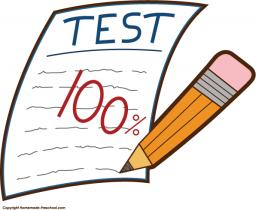Did you find an error or inaccuracy? Feel free to write us. Thank you!Tips to related online calculators
Do you have a linear equation or system of equations and looking for its solution? Or do you have a quadratic equation?

## Related math problems and questions:

• StudentsStudents solve word problems. They received 3 points for each correctly solved task. Four points were deducted for a task that was not solved or incorrectly solved. Tomas solved a total of 15 tasks and scored 24 points. How many tasks did he solve correct
• The math testThe math test contains 20 problems. For each correctly solved problem, the solver gets 3 points, for each incorrectly solved or unsolved problem, 2 points are deducted. Ondrej got 25 points. How many problems did he solve correctly?
• Math testObelix filled a mathematical test in which he answered 25 questions. For every correct answer, he received 5 points, for each bad answer he had 3 points deducted. Obelix gained 36% of all points in the test. How many questions did he solve correctly?
• SubjectsStudent makes a mathematical task for five minutes and the task from biology in twelve minutes. Another day he have homework in math and biology which consists of eleven tasks together. To do the job took hour and a half. How many tasks maked in each subj
• Petra's testThe answer will be given 8 marks, and the wrong answer will be deducted 3 marks. The total number of marks obtained by the school is 36. If Petra answered six questions correctly,   how many questions were answered incorrectly by the student from the scho
• TV competitionIn the competition, 10 contestants answer five questions, one question per round. Anyone who answers correctly will receive as many points as the number of competitors answered incorrectly in that round. After the contest, one of the contestants said: We
• Math testIn mathematics, there were 25 problems of three kinds: light 2 points, medium 3 points, heavy 5 points, the best score is 84 points. How many points did Jane have when she solved all the easy examples, half medium and one-third difficult?
• Math testStudents wrote a math test. The average number of points obtained by them was 64. Another student wrote this test for 80 points. If the teacher added his result to the original ones, the total average of all students would be 65. How many students origina
• PotatoesDaniela and Michael would jointly dug potatoes for 7.5 hours. But if Daniela was working alone she would take 2.5 hours more as if he were working with Michael. Determine how much for the work done by Michael himself and how much Daniela herself.
• Football league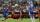In the 2nd football league plays each of the 16 participants with each opponent twice. For every victory gets 3 points, 1 point for a draw, a defeat none. After the competition was team with 54 points and 12 defeats at 9th place. How many times have won?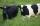Grass grows in the meadow equally fast and evenly. It is known that 99 cows graze meadow for 14 days and 95 cows by 22 days. How many cows graze meadow for 77 days?The teacher prepared a test with ten questions. The student can choose one correct answer from the four (A, B, C, D). The student did not get a written exam at all. What is the probability that: a) He answers half correctly. b) He answers all correctly. c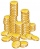John has 540 CZK, but he has 10 CZK coins and 20 CZK coins only, we knows that he has 10CZK coins 7times more that 20CZK coins, how many 10CZK coin and how many 20CZK coins he saved?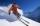the ski course attends 80 pupils. They live in triple and quadruple rooms. Count if rooms are the 23. How many are triple and quadruple rooms?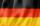Of the 35 students of the class were 7 on holiday in Germany and just as much in Italy. 5 students visited Austria. In none of these countries was 21 students, all three visited by one student. In Italy and Austria were 2 students and in Austria and Germa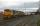The train carries 525 tons of limestone in 29 wagons. Wagons are 15 tonne and 20 tons. How many is 15 ton and how many is 20 ton wagons?In the yard were chickens and rabbits. Together they had 18 heads and 56 legs. How many chickens and how many rabbits were in the yard?